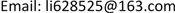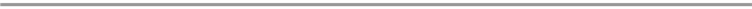﻿ Fp上一类MDS符号对码的构造 The Construction of a Class of MDS Symbol-Pair Codes over Fp

Pure Mathematics
Vol. 10  No. 02 ( 2020 ), Article ID: 34161 , 6 pages
10.12677/PM.2020.102009

The Construction of a Class of MDS Symbol-Pair Codes over Fp

Shaopei Li, Xilin Tang

School of Mathematics, South China University of Technology, Guangzhou GuangdongReceived: Jan. 17th, 2020; accepted: Feb. 4th, 2020; published: Feb. 11th, 2020ABSTRACT

Symbol-pair codes are designed to protect against pair error in data reading. The pair-distance is an important parameter to measure the error correction ability of the symbol pair in the symbol pair reading channel. MDS symbol pair codes are the best symbol pair codes with the largest symbol pair distance when the length and dimension of the symbol pair codes are constant. One of the important problems of symbol-pair codes is to construct MDS symbol-pair codes with a large code length and a large minimum pair-distance. In this paper, we analyze the method of characterizing pair-distance by repeated-root cyclic codes and construct a new class of MDS symbol-pair codes with different parameters and larger symbol pair-distance.

Keywords:MDS Symbol-Pair Codes, Minimum Pair-Distance, Constacyclic CodesFp上一类MDS符号对码的构造1. 研究背景

2. 预备知识

$x=\left({x}_{0},{x}_{1},\cdots ,{x}_{n-1}\right)\in {\Sigma }^{n}$，那么x对应的符号对向量为

$\pi \left(x\right)=\left[\left({x}_{0},{x}_{1}\right),\left({x}_{1},{x}_{2}\right),\cdots ,\left({x}_{n-1},{x}_{0}\right)\right]$, (1.1)

$\pi \left(x\right)$ 的每一个分量 $\left({x}_{i},{x}_{i+1}\right)$ 被称为一个符号对，显然 $\pi \left(x\right)$ 由x唯一确定。对 $\forall \left(a,b\right),\left(c,d\right)\in \Sigma ×\Sigma$，有 $\left(a,b\right)=\left(c,d\right)$ 当且仅当 $a=b$$c=d$。设 $x=\left({x}_{0},{x}_{1},\cdots ,{x}_{n-1}\right)$$y=\left({y}_{0},{y}_{1},\cdots ,{y}_{n-1}\right)\in {\Sigma }^{n}$，定义

${d}_{P}\left(x,y\right)={d}_{H}\left(\pi \left(x\right),\pi \left(y\right)\right)$ (1.2)

${d}_{P}\left(x\right)=|\left\{{x}_{i}\ne 0|{x}_{i}\in x\right\}|$, (1.3)

$gap\left(x\right)=|\left\{0\le i\le n-1|{x}_{i}\in {F}_{q}^{*},{x}_{i+1}=0\right\}|$, (1.4)

${d}_{P}\left(x\right)=w{t}_{H}\left(x\right)+gap\left(x\right)$. (1.5)

${d}_{P}\left(C\right)=\mathrm{min}\left\{{d}_{P}\left(x,y\right):\forall x,y\in C,x\ne y\right\}$.(1.6)

$\Omega =\left\{1+rj|0\le j\le n-1\right\}$,(1.7)

${C}_{S}=\left\{s,sq,s{q}^{2},\cdots \right\}\left(\mathrm{mod}nr\right)$,(1.8)

$g\left(x\right)=\underset{s\in A}{\prod }\underset{i\in {C}_{s}}{\prod }\left(x-{\delta }^{i}\right)$, (1.9)

$g\left(x\right)=\underset{i=1}{\overset{k}{\prod }}{m}_{i}{\left(x\right)}^{{e}_{i}}$,(1.10)

${D}_{t}=〈{g}_{t}\left(x\right)〉$,(1.11)

${d}_{H}\left({D}_{t}\right)=\left\{\begin{array}{l}\infty ,\text{\hspace{0.17em}}\text{\hspace{0.17em}}{g}_{t}\left(x\right)={x}^{l}-{\lambda }_{0}\\ 1\text{ },\text{\hspace{0.17em}}\text{\hspace{0.17em}}\text{\hspace{0.17em}}{g}_{t}\left(x\right)=1\end{array}$, (1.12)

${d}_{H}\left(C\right)=\mathrm{min}\left\{{P}_{t}\cdot {d}_{H}\left({D}_{t}\right)|0\le t\le {p}^{s}-1\right\}$ (1.13)

3. Fp上长为2p的MDS符号对码

Case1: $w{t}_{H}\left(c\right)=\text{5}$$gap\left(c\right)=1$

Case2: $w{t}_{H}\left(c\right)=\text{4}$$gap\left(c\right)=2$

(i) $c\left(x\right)={c}_{0}+{c}_{1}x+{c}_{i}{x}^{i}+{c}_{i+1}{x}^{i+1},\left(3\le i\le 2p-2\right)$，其中 ${c}_{0},{c}_{1},{c}_{i},{c}_{i+1}\in {F}_{q}^{*}$，由于 $g\left(x\right)|c\left(x\right)$，那么

$\left\{\begin{array}{l}{c}_{0}+{c}_{1}+{c}_{i}+{c}_{i+1}=0\\ {c}_{1}+i{c}_{i}+\left(i+1\right){c}_{i+1}=0\\ i\left(i-1\right){c}_{i}+\left(i+1\right)i{c}_{i+1}=0\\ {c}_{0}-{c}_{1}+{\left(-1\right)}^{i}{c}_{i}+{\left(-1\right)}^{i+1}{c}_{i+1}=0\\ {c}_{1}+i{\left(-1\right)}^{i-1}{c}_{i}+\left(i+1\right){\left(-1\right)}^{i}{c}_{i+1}=0\end{array}$ (2.1)

$i\left[1-{\left(-1\right)}^{i-1}\right]{c}_{i}+\left(i+1\right)\left[1-{\left(-1\right)}^{i}\right]{c}_{i+1}=0$, (2.2)

$\left(i+1\right){c}_{i+1}=0$, (2.3)

$i+1\ne 0$，那么 ${c}_{i+1}=0$，推出矛盾；

$i+1=0$，由(2.1)中第三个方程可得： $2{c}_{i}=0$，即 ${c}_{i}=0$，推出矛盾；

(ii) $c\left(x\right)={c}_{0}+{c}_{1}x+{c}_{2}{x}^{2}+{c}_{i}{x}^{i},\left(4\le i\le 2p-1\right)$，其中 ${c}_{0},{c}_{1},{c}_{2},{c}_{i}\in {F}_{q}$，由于 $g\left(x\right)|c\left(x\right)$，那么

$\left\{\begin{array}{l}{c}_{0}+{c}_{1}+{c}_{2}+{c}_{i}=0\hfill \\ {c}_{1}+2{c}_{2}+i{c}_{i}=0\hfill \\ 2{c}_{2}+i\left(i-1\right){c}_{i}=0\hfill \\ {c}_{0}-{c}_{1}+{c}_{2}+{\left(-1\right)}^{i}{c}_{i}=0\hfill \\ {c}_{1}-2{c}_{2}+i{\left(-1\right)}^{i-1}{c}_{i}=0\hfill \end{array}$ (2.4)

$2{c}_{1}+\left[1-{\left(-1\right)}^{i}\right]{c}_{i}=0$, (2.5)

${c}_{1}+{c}_{i}=0$,(2.6)

$2{c}_{2}+\left(i-1\right){c}_{i}=0$, (2.7)

${C}_{i}=\left\{c\in C:w{t}_{H}=i\right\}$, (2.8)

$4\le i\le 10$，C中码字按照Hamming距离分类处理如下：

1) $|{C}_{4}|=40$${C}_{4}$ 中的码字具有4中的形式： $\left(\text{0102030400}\right)$$\left(\text{0204010300}\right)$$\left(\text{0301040200}\right)$$\left(0\text{4}0\text{3}0\text{2}0\text{1}00\right)$，可知 $\forall c\in {C}_{4}$$gap\left(c\right)=4$，从而 ${d}_{P}\left(c\right)=8$

2) $|{C}_{5}|=8$${C}_{5}$ 中的码字具有4中的形式： $\left(\text{0101010101}\right)$$\left(\text{0202020202}\right)$$\left(\text{0303030303}\right)$$\left(\text{0404040404}\right)$，可知 $\forall c\in {C}_{5}$$gap\left(c\right)=5$，从而 ${d}_{P}\left(c\right)=10$

3) $|{C}_{6}|=400$$\exists {c}^{\prime }=\left(2,3,1,4,2,3,0,0,0,0\right)\in {C}_{6}$ s.t. $gap\left({c}^{\prime }\right)=1$，那么 ${d}_{P}\left({c}^{\prime }\right)=7$，从而 $\forall c\in {C}_{6}$${d}_{P}\left(c\right)\ge 7$，此处的“=”能够成立；

4) 如果 $w{t}_{H}\left(c\right)\ge 7$，那么 ${d}_{P}\left(c\right)\ge w{t}_{H}\left(c\right)+gap\left(c\right)\ge 7$

${C}_{i}=\left\{c\in C:w{t}_{H}=i\right\}$,(2.9)

$4\le i\le 10$，C中码字按照Hamming距离分类处理如下：

1) $|{C}_{4}|=420$，对 $\forall c\in {C}_{\text{4}}$$gap\left(c\right)=4$，此时 ${d}_{P}\left(c\right)=8$

2) $|{C}_{5}|=756$，对 $\forall c\in {C}_{\text{5}}$$gap\left(c\right)=5$，此时 ${d}_{P}\left(c\right)=10$

3) $\exists {c}^{″}=\left(1,6,5,2,1,6,0,0,0,0,0,0,0,0\right)\in {C}_{6}$，s.t. $gap\left({c}^{″}\right)=1$，那么 ${d}_{P}\left({c}^{″}\right)=7$，从而 $\forall c\in {C}_{6}$${d}_{P}\left(c\right)\ge 7$，此处的“=”能够成立；

4) 如果 $w{t}_{H}\left(c\right)\ge 7$，那么 ${d}_{P}\left(c\right)\ge w{t}_{H}\left(c\right)+gap\left(c\right)\ge 7$

4. 结论

The Construction of a Class of MDS Symbol-Pair Codes over Fp[J]. 理论数学, 2020, 10(02): 49-54. https://doi.org/10.12677/PM.2020.102009

1. 1. Cassuto, Y. and Blaum, M. (2010) Codes for Symbol-Pair Read Channels. Proceedings of IEEE International Symposium on Information Theory, Austin, TX, USA, June 2010, 988-992.https://doi.org/10.1109/ISIT.2010.5513753

2. 2. Cassuto, Y. and Blaum, M. (2011) Codes for Symbol-Pair Read Channels. IEEE Transactions on Information Theory, 57, 8011-8020.https://doi.org/10.1109/TIT.2011.2164891

3. 3. Chee, Y.M., Ji, L., Kiah, H.M., et al. (2013) Maximum Distance Separable Codes for Symbol-Pair Read Channels. IEEE Transactions on Information Theory, 59, 7259-7267. https://doi.org/10.1109/TIT.2013.2276615

4. 4. Kai, X., Zhu, S. and Li, P. (2015) A Construction of New MDS Symbol-Pair Codes. IEEE Transactions on Information Theory, 61, 5828-5834.https://doi.org/10.1109/TIT.2015.2481889

5. 5. Chen, B., Lin, L. and Liu, H. (2016) Constacyclic Symbol-Pair Codes: Lower Bounds and Optimal Constructions. IEEE Transactions on Information Theory, 63, 7661-7666.https://doi.org/10.1109/TIT.2017.2753250

6. 6. Kai, X., Zhu, S., Zhao, Y., Luo, H. and Chen, Z. (2018) New MDS Symbol-Pair Codes from Repeated-Root Codes. IEEE Communications Letters, 22, 462-465.https://doi.org/10.1109/LCOMM.2018.2791422

7. 7. Cassuto, Y. and Blaum, M. (2011) Codes for Symbol-Pair Read Channels. IEEE Transactions on Information Theory, 57, 8011-8020.https://doi.org/10.1109/TIT.2011.2164891